• 线性模型：试图学得一个通过属性的线性组合来进行预测的函数，即 3.1 用向量形式写成： 3.2 2.线性回归 对于给定数据集D={(x1,y1),(x2,y2),(x3,y3),.....,(xm,ym)},其中 x1=(x1;x2;...;xd),yi...
1. 基本形式

线性模型：试图学得一个通过属性的线性组合来进行预测的函数，即3.1

用向量形式写成：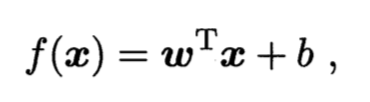3.2

2.线性回归

对于给定数据集D={(x1,y1),(x2,y2),(x3,y3),.....,(xm,ym)},其中 x1=(x1;x2;...;xd),yi ∈ R,"线性回归"试图学得一个线性模型以尽可能准确的预测实值输出标记。
我们先考虑一种最简单的情形：输入属性数目只有一个即image.png

线性回归试图学得：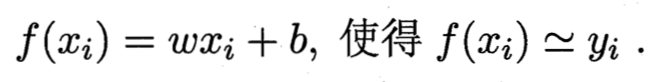image.png

如何确定w和b呢？显然，关键在于如何衡量f(x)与y之间的差别，之前介绍过，均方误差是回归任务常用的性能度量,因此我们试图让均方误差最小化，即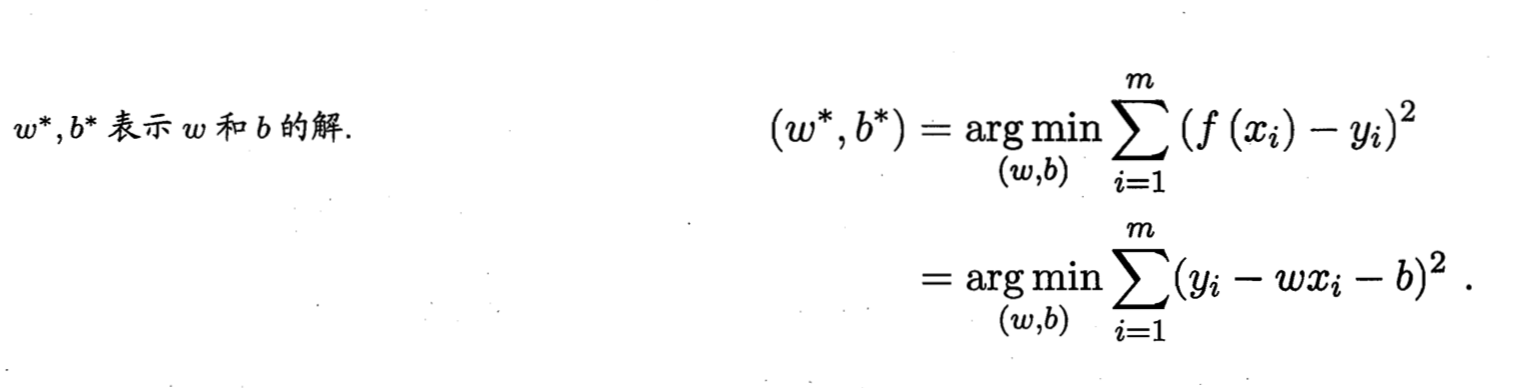image.png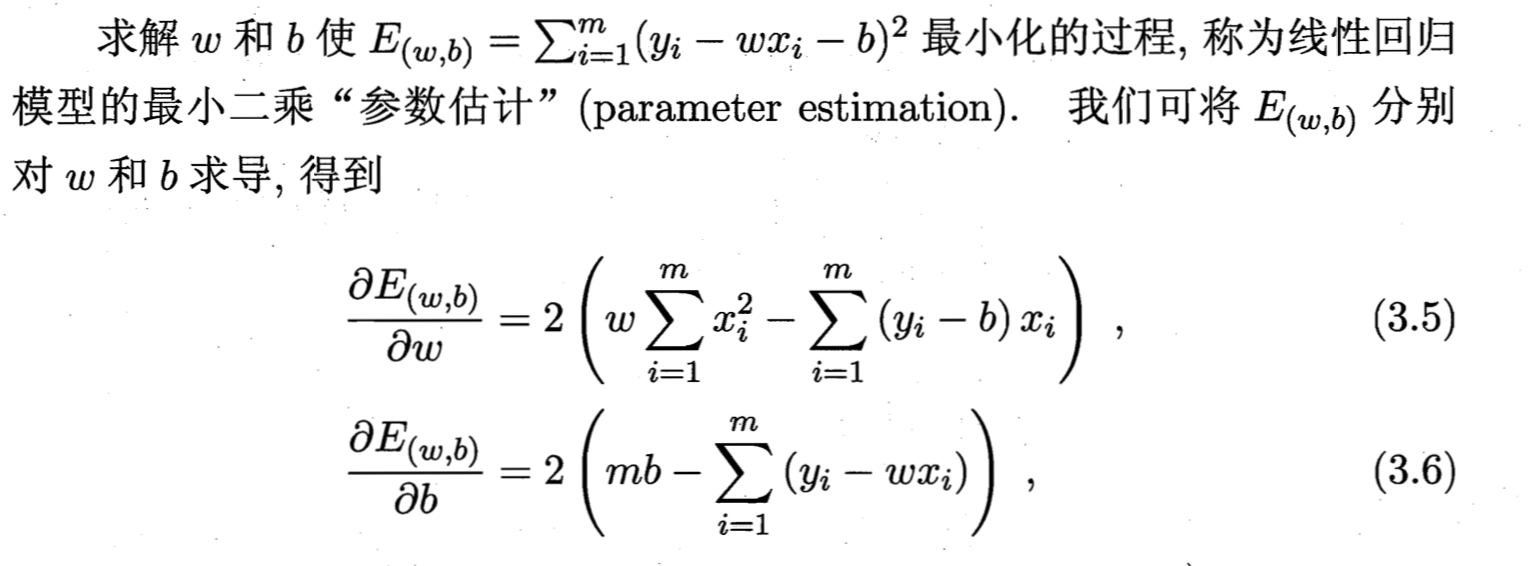image.png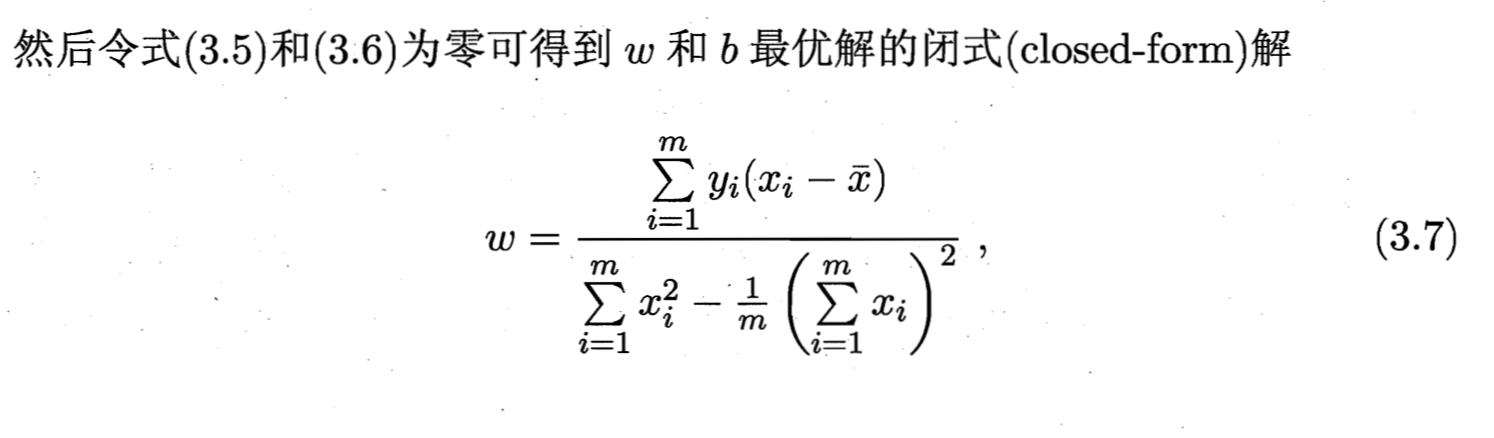image.png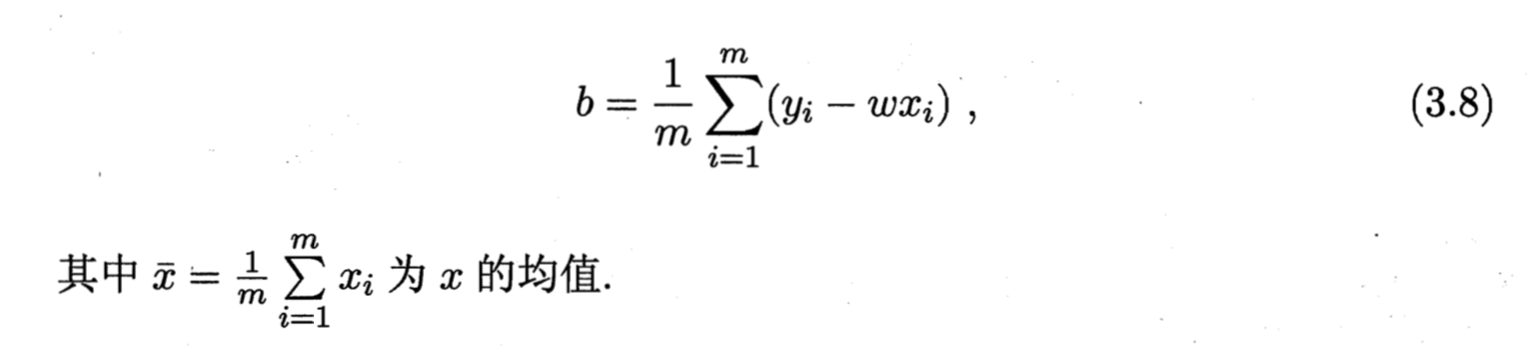image.png

展开全文• 例：尝试一个线性模型 让我们来使用目前为止学到的概念—Tensor，Variable，和 GradientTape—来创建和训练一个简单的模型。一般需要下面这些步骤： 定义模型 定义损失函数 获取训练数据 通过训练数据运行模型，使用...
例：尝试一个线性模型
定义模型
定义损失函数
获取训练数据
通过训练数据运行模型，使用 “optimizer” 来调整变量以满足数据
在这个教程中，我们使用一个简单线性模型作为示例：f(x) = x * W + b，有2个变量- W 和 b。另外，我们会生成数据让训练好的模型满足 W = 3.0 和 b = 2.0。
定义模型
定义一个简单的类封装变量和计算
class Model(object):
def __init__(self):
# 初始化变量值为(5.0, 0.0)
# 实际上，这些变量应该初始化为随机值
self.W = tf.Variable(5.0)
self.b = tf.Variable(0.0)

def __call__(self, x):
return self.W * x + self.b

model = Model()

assert model(3.0).numpy() == 15.0

定义损失函数
损失函数用来衡量在给定输入的情况下，模型的预测输出与实际输出的偏差。我们这里使用标准 L2 损失函数。
def loss(predicted_y, desired_y):
return tf.reduce_mean(tf.square(predicted_y - desired_y))

获取训练数据
我们来生成带噪声的训练数据。
TRUE_W = 3.0
TRUE_b = 2.0
NUM_EXAMPLES = 1000

inputs  = tf.random.normal(shape=[NUM_EXAMPLES])
noise   = tf.random.normal(shape=[NUM_EXAMPLES])
outputs = inputs * TRUE_W + TRUE_b + noise

在训练模型之前，我们来看看当前的模型表现。我们绘制模型的预测结果和训练数据，预测结果用红色表示，训练数据用蓝色表示。
import matplotlib.pyplot as plt

plt.scatter(inputs, outputs, c='b')
plt.scatter(inputs, model(inputs), c='r')
plt.show()

print('Current loss: '),
print(loss(model(inputs), outputs).numpy())


定义训练循环
我们已经定义了网络模型，并且获得了训练数据。现在对模型进行训练，采用梯度下降的方式，通过训练数据更新模型的变量（W 和 b）使得损失量变小。梯度下降中有很多参数，通过 tf.train.Optimizer 实现。我们强烈建议使用这些实现方式，但基于通过基本规则创建模型的精神，在这个特别示例中，我们自己实现基本的数学运算。
def train(model, inputs, outputs, learning_rate):
current_loss = loss(model(inputs), outputs)
dW, db = t.gradient(current_loss, [model.W, model.b])
model.W.assign_sub(learning_rate * dW)
model.b.assign_sub(learning_rate * db)

训练
最后，我们对训练数据重复地训练，观察 W 和 b 是怎么变化的。
model = Model()
# 收集 W 和 b 的历史数值，用于显示
Ws, bs = [], []
epochs = range(10)
for epoch in epochs:
Ws.append(model.W.numpy())
bs.append(model.b.numpy())
current_loss = loss(model(inputs), outputs)

train(model, inputs, outputs, learning_rate=0.1)
print('Epoch %2d: W=%1.2f b=%1.2f, loss=%2.5f' %
(epoch, Ws[-1], bs[-1], current_loss))

# 显示所有
plt.plot(epochs, Ws, 'r',
epochs, bs, 'b')
plt.plot([TRUE_W] * len(epochs), 'r--',
[TRUE_b] * len(epochs), 'b--')
plt.legend(['W', 'b', 'true W', 'true_b'])
plt.show()



展开全文• 线性模型 Python 实现 import numpy as np from sklearn import linear_model class LinearRegression: def __init__(self): self.w = None # 要训练的参数 self.n_features = None # 特征的个数 def fit(s
哔哩哔哩视频地址 https://www.bilibili.com/video/BV1s54y1U7sG
线性模型 Python 实现
import numpy as np
from sklearn import linear_model

class LinearRegression:
def __init__(self):
self.w = None  # 要训练的参数
self.n_features = None   # 特征的个数

def fit(self,X,y):    # 计算权重 W
"""
w=(X^TX)^{-1}X^Ty
"""
assert isinstance(X,np.ndarray) and isinstance(y,np.ndarray)
assert X.ndim==2 and y.ndim==1    # assert 断言，就是说后面的条件成立时执行下面代码，不满足时返回错误
assert y.shape==X.shape
n_samples = X.shape            #样本数量
self.n_features = X.shape      #特征个数，X.shape是个元组，元组的第二位代表列，也就是特征个数
extra = np.ones((n_samples,))
X = np.c_[X,extra]                #是在行方向扩展连接两个矩阵，就是把两矩阵左右相加，要求行数相等，相当于 hstack https://blog.csdn.net/qq_43657442/article/details/108030183
if self.n_features < n_samples:
self.w = np.linalg.inv(X.T.dot(X)).dot(X.T).dot(y)  # 其实就是X的广义逆乘Y，得到权重，详解见https://blog.csdn.net/qq_43657442/article/details/108032355
else:
raise ValueError('dont have enough samples')

def predict(self, X):
n_samples=X.shape
extra = np.ones((n_samples,))  #产生一个二维数组，用这样的方式代表这个数组可行向量可列向量
X = np.c_[X, extra]
if self.w is None:
raise RuntimeError('cant predict before fit')
y_=X.dot(self.w)
return y_

if __name__ == '__main__':
X = np.array([[1.0,0.5,0.5],[1.0,1.0,0.3],[-0.1,1.2,0.5],[1.5,2.4,3.2],[1.3,0.2,1.4]])
y = np.array([1,0.5,1.5,2,-0.3])
lr = LinearRegression()
lr.fit(X,y)
X_test = np.array([[1.3,1,3.2],[-1.2,1.2,0.8]])
y_pre = lr.predict(X_test)
print(y_pre)

sklearn_lr = linear_model.LinearRegression()
sklearn_lr.fit(X,y)
sklearn_y_pre = sklearn_lr.predict(X_test)
print(sklearn_y_pre)

ridge_reg = linear_model.Ridge(alpha=0.05, solver='lsqr')  # 岭回归，具有L2正则化的线性最小二乘法回归模型，损失函数是线性最小二乘函数，而正则化由l2-范数给出, alpha 是正则化的罚系数
ridge_reg.fit(X, y)
ridge_y_pre=ridge_reg.predict(X_test)
print(ridge_y_pre)


机器学习 线性模型里面的线性回归


展开全文• 参考：周志华机器学习第三章1. 基本形式给定由d个属性描述的示例x=(x1;x2;...;xd)x=(x_1;x_2;...;x_d)，其中xi是x在第i个属性上的取值，线性模型试图学得一个通过属性的线性组合来进行预测函数，即 f(x)=w1x1+w2x2+...
参考：周志华机器学习第三章

1. 基本形式

给定由d个属性描述的示例x=(x1;x2;...;xd)<!--//--><![CDATA[//><!--
x=(x_1;x_2;...;x_d)
//--><!]]>，其中xi是x在第i个属性上的取值，线性模型试图学得一个通过属性的线性组合来进行预测函数，即 f(x)=w1x1+w2x2+...+wdxd+b<!--//--><![CDATA[//><!--
f(x)=w_1x_1+w_2x_2+...+w_dx_d+b
//--><!]]>
一般用向量形式写成 f(x)=wtx+b<!--//--><![CDATA[//><!--
f(x)=w^tx+b
//--><!]]>其中w=(w1;w2;...;wd)<!--//--><![CDATA[//><!--
w=(w_1;w_2;...;w_d)
//--><!]]>.w和b学得之后，模型就得以确定。
线性模型的特点：

形式简单
易于建模，非线性模型可在线性模型基础上引入层次结构或高维映射而得
具有很好的可解释性
2. 线性回归

基本形式

给定数据集D=(x1,y1),(x2,y2),...,(xm,ym)<!--//--><![CDATA[//><!--
D={(x_1,y_1),(x_2,y_2),...,(x_m,y_m)}
//--><!]]>，其中xi=(xi1;xi2;xi3;...;xid)<!--//--><![CDATA[//><!--
x_i=(x_{i1};x_{i2};x_{i3};...;x_{id})
//--><!]]>，线性回归试图学得一个线性模型以尽可能准确地预测实值输出标记。最简单的情形是输入属性的数目只有一个时，此时D={(xi,yi)}mi=1,xi∈R<!--//--><![CDATA[//><!--
D=\{(x_i,y_i)\}_{i=1}^m,x_i \in R
//--><!]]>. 周志华老师教材中使用的数据集如下：

编号
色泽
根蒂
敲声
纹理
脐部
触感
密度
含糖率
好瓜
1
青绿
蜷缩
浊响
清晰
凹陷
硬滑
0.697
0.46
是
2
乌黑
蜷缩
沉闷
清晰
凹陷
硬滑
0.774
0.376
是
3
乌黑
蜷缩
浊响
清晰
凹陷
硬滑
0.634
0.264
是
4
青绿
蜷缩
沉闷
清晰
凹陷
硬滑
0.608
0.318
是
5
浅白
蜷缩
浊响
清晰
凹陷
硬滑
0.556
0.215
是
6
青绿
稍蜷
浊响
清晰
稍凹
软粘
0.403
0.237
是
7
乌黑
稍蜷
浊响
稍糊
稍凹
软粘
0.481
0.149
是
8
乌黑
稍蜷
浊响
清晰
稍凹
硬滑
0.437
0.211
是
9
乌黑
稍蜷
沉闷
稍糊
稍凹
硬滑
0.666
0.091
否
10
青绿
硬挺
清脆
清晰
平坦
软粘
0.243
0.267
否
11
浅白
硬挺
清脆
模糊
平坦
硬滑
0.245
0.057
否
12
浅白
蜷缩
浊响
模糊
平坦
软粘
0.343
0.099
否
13
青绿
稍蜷
浊响
稍糊
凹陷
硬滑
0.639
0.161
否
14
浅白
稍蜷
沉闷
稍糊
凹陷
硬滑
0.657
0.198
否
15
乌黑
稍蜷
浊响
清晰
稍凹
软粘
0.36
0.37
否
16
浅白
蜷缩
浊响
模糊
平坦
硬滑
0.593
0.042
否
17
青绿
蜷缩
沉闷
稍糊
稍凹
硬滑
0.719
0.103
否
注意到xi<!--//--><![CDATA[//><!--
x_i
//--><!]]>的取值范围是实数，因此需要将“青绿”、“平坦”等属性值转化为实值。对于离散属性，若属性值间存在“序”关系，则通过连续化将其转化为连续值，如身高的高、低可转化为{1.0,0.0}<!--//--><![CDATA[//><!--
\{1.0, 0.0\}
//--><!]]>；若属性值间不存在序关系，则通常将k个属性值转化为k维向量，如将属性“瓜类”的取值“西瓜、南瓜、黄瓜”转化为(0,0,1),(0,1,0),(1,0,0)。
线性回归试图学得f(xi)=wxi+b<!--//--><![CDATA[//><!--
f(x_i)=wx_i+b
//--><!]]>，使得f(xi)≈yi<!--//--><![CDATA[//><!--
f(xi) \approx y_i
//--><!]]>
确定w和b的关键在于如何衡量f(x)与y之间的差距。这就要用到性能度量。

度量

回归任务最常使用的性能度量是均方误差。 (w∗,b∗)=argmin(w,b)∑mi=1(f(xi)−yi)2=argmin(w,b)∑mi=1(yi−wxi−b)2<!--//--><![CDATA[//><!--
(w^*,b^*)= \underset{(w,b)}{argmin}\sum_{i=1}^m(f(x_i)-y_i)^2=\underset{(w,b)}{argmin}\sum_{i=1}^m(y_i-wx_i-b)^2
//--><!]]>

均方误差也叫作平方损失（square loss），对应了常见的欧氏距离，基于均方误差最小化进行求解的方法称为“最小二乘法（least square method）。在线性回归中，最小二乘法就是试图找到一条直线，使所有样本到直线上的欧氏距离之和最小。

参数估计

求解w和b使E(w,b)=∑mi=1(yi−wxi−b)2<!--//--><![CDATA[//><!--
E_{(w,b)}=\sum_{i=1}^m(y_i-wx_i-b)^2
//--><!]]>最小化的过程，称为线性回归模型的最小二乘参数估计。将E(w,b)<!--//--><![CDATA[//><!--
E_{(w,b)}
//--><!]]>分别对w和b求导并使其为零可得到w和b最优解的闭式解。 w=∑mi=1yi(xi−x¯)∑mi=1x2i−1m∑mi=1xi2<!--//--><![CDATA[//><!--
w=\frac {\sum_{i=1}^my_i(x_i-\overline x)} {\sum_{i=1}^mx_i^2-\frac 1 m {\sum_{i=1}^mx_i}^2}
//--><!]]>b=1m∑mi=1(yi−wxi)<!--//--><![CDATA[//><!--
b=\frac 1 m \sum_{i=1}^m(y_i-wx_i)
//--><!]]>  其中x¯<!--//--><![CDATA[//><!--
\overline x
//--><!]]>为x的均值
展开全文笔记
• 在目前的机器学习领域中，最常见的三种任务就是：回归分析、分类分析、聚类分析。那么什么是回归呢？回归分析是一种预测性的建模技术...线性回归拟合一个带系数的线性模型，以最小化数据中的观测值与线性预测值之间...
• 1. 机器学习（一）线性回归 给定数据集 D={(x1x_1x1​,y1y_1y1​),(x2x_2x2​,y2y_2y2​),…,(xmx_mxm​,ymy_mym​)},其中xi=(xi1;xi2;...;xid),yi∈Rx_i = (x_{i1};x{_i2};...;x_{id}),y_i \in Rxi​=(xi1​;xi​2...单变量 matlab
• 机器学习线性回归模型Linear Regression is one of the fundamental supervised-machine learning algorithm. While it is relatively simple and might not seem fancy enough when compared to other Machine ...人工智能 python 深度学习 逻辑回归
• 机器学习线性模型1.一元线性回归模型2.多元线性回归模型逻辑回归模型（对数几率回归）Logistic回归模型的实践 线性回归也就是可以用一条直线来拟合一个点集。博主大概总结下机器学习线性模型，以及主要的公式推...
• 机器学习线性回归模型，用梯度下降算法实现的线性回归，logistic回归的模型以及实例分析。包含上课时用的PDF、word以及PPT文档
• 文章目录线性模型1.基本形式1.1线性模型2.线性回归2.1线性回归2.2多回归任务：广义线性回归对数线性回归对数几率线性回归softmax回归线性判别回归局部线性判别分析特征选择多分类问题 线性模型 1.基本形式 1.1线性...
• 每一本机器学习的书、统计学方法的书在前面的某个章节，一定会讲到线性模型。因为它几乎是一切机器学习、深度学习算法的基础。写这篇，就是想从一个菜鸟的视角，尝试讲清楚线性模型，以及个人学习过程中的体会。 ...
• 机器学习线性模型(Generalized Linear Models)一 本篇主要针对于Ordinary Least Squares，周志华的机器学习中关于线性模型章节3.2首先提到了对数据数字化的过程，原文是：对离散属性，若属性值间存在“序”关系，...
• 机器学习线性模型 乐川科技有限公司CEO，人工智能培训讲师，专业从事机器学...
• 一、线性模型的基本形式 给定由d个属性描述的示例X=(X1;X2;X3……;Xd)，其中Xi是X的X在第i个属性上的取值...线性回归是一种监督学习下的线性模型，线性回归试图给定数据集中学习一个线性模型来较好的预测输出(可视...
• 线性回归是一种监督学习下的线性模型，线性回归试图从给定数据集中学习一个线性模型来较好的预测输出（可视为：新来一个不属于D的数据，我们只知道他的x，要求预测y，D如下表示）。 首先我们还是给定数据集的严格...逻辑回归...